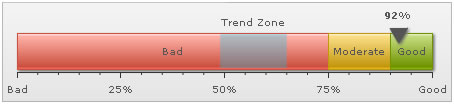You are viewing documentation for an older version. For current documentation - click here.

The linear gauge allows you to add the following trend elements on the chart:

Here we'll see how to add these and configure the same.

To add a simple trend point (say last year's customer satisfaction index as 79%), you'll need to add the following to XML:

`<trendpoints>  <point startValue="79" displayValue="Previous" color="666666" thickness="2" alpha="100"/></trendpoints>`
```"trendpoints": {
"point": [
{
"startvalue": "79",
"displayvalue": "Previous",
"color": "666666",
"thickness": "2",
"alpha": "100"
}
]
}```

Here, we've first added the <trendpoints> element, which can contain any number of trend-points for the chart. Now, for each trend point, you need to add a <point> element with startValue/endValue as the value and other cosmetic properties defined.

The above trend point will show up on the chart as under: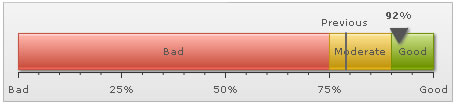You can have the following properties for <point> element:

 Attribute Name Type / Range Description startValue Number The starting value for the trend-point. Say, if you want to plot a trend zone from value 102 to 109, the startValue will be 102. endValue Number The ending value for the trend-point. Say, if you want to plot a trend-zone from value 102 to 109, the endValue will be 109. displayValue String Display label for the trend-point. If you do not specify a value, the chart displays the numeric value. showOnTop Boolean (0/1) Whether to display the value on top? color Color Code Color of the trend point and its associated text. alpha Number (0-100) Alpha of the trend line. dashed Boolean (0/1) If you're showing the trend point as line, this attribute lets you control whether the line will be plotted as dashed? dashLen Number (pixels) Length of each dash, if trend-point is plotted as dashed line. dashGap Number (pixels) Length of each dash gap, if trend-point is plotted as dashed line. useMarker Boolean (0/1) You can opt to show a triangular marker for each trend-point by setting this attribute to 1. markerColor Color Code Fill color of marker. markerBorderColor Color Code Border color of marker. markerRadius Number (pixels) Radius of the marker. markerTooltext String You can define tooltip for marker using this marker.

The line can also be drawn as dashed one by setting:

`<point ... dashed='1' dashLen='3' dashGap='3' ..>`
`"point": [ {... "dashed": "1", "dashlen": "3", "dashgap": "3" ...} ] }`

This will result in: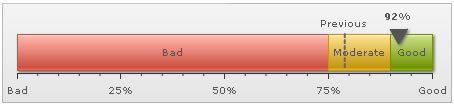You can opt to show trend label below the gauge using:

`<point ... showOnTop='0' ...>`
`"point": [ {... "showontop": "0" ...} ] }`

This will result in: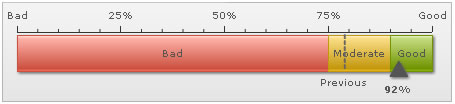If you wish to increase the space between trend line and its value, you can set it in:

<chart ... trendValueDistance='25' ..> (or a value in pixels)

Showing trend-markers

For each trend point, you can also show a triangular marker using:

`<point startValue='79' displayValue='Previous' color='666666' useMarker='1' markerColor='F1f1f1' markerBorderColor='666666' markerRadius='7'/>`
``` "point": [ { "startvalue": "79", "displayvalue": "Previous", "color": "666666", "usemarker": "1", "markercolor": "F1F1F1",
"markerbordercolor": "666666", "markerradius": "7" } ] ```

This will result in: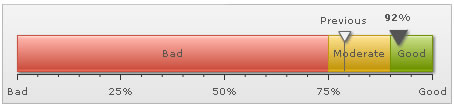You can also add a tooltip to this marker using:

`<point ... markerTooltext='Previous year index: 79%'   ...>`
`"point": [ {... "markertooltext": "Previous year index: 79%" ...} ] `

This will result in: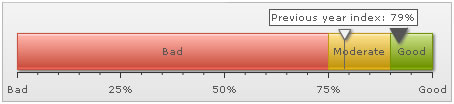Creating trend-zones

You can also create trend-zone to represent a range of values, instead of a single value. Shown below is a simple XML:

`<point startValue='49' endValue='65' displayValue='Trend Zone' color='00CCFF' alpha='30' />`
`"point": [ { "startvalue": "49", "endvalue": "65", "displayvalue": "Trend Zone", "color": "00CCFF", "alpha": "30"} ] `

Here, we've added both startValue and endValue to create a trend zone. Also, we've specified the cosmetics. This will result in: